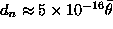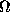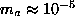The Net Advance of Physics: The Nature of Dark Matter, by Kim Griest -- Section 5.

Next: Search for Wimps Up: Distribution Previous: Thermal Relics (Wimps)

# Non-thermal Relics as Dark Matter (Axions)

The best example of a non-thermal particle dark matter candidate

is the axion . Actually, thermal axions are produced in the

standard way, but if such axions existed in numbers sufficient to

make up the dark matter, they would have lifetimes too short to still

be around in quantity. However, there is another, more important,

production mechanism for axions in the early Universe.

The axion arises because the QCD Lagrangian contains a termwhere G is the gluon field strength. This term predicts an electric

dipole moment of the neutron of is.

Experimentally, however, the neutron dipole moment,

which means. The question is why does thisparameter

have such a small value, when it naturally would have a value near

unity? This is the strong CP problem, and one way to resolve this

problem is to introduce a new Peccei-Quinn symmetry which

predicts a new particle - the axion. The P-Q symmetry forcesat low temperatures today, but in the early Universe, the axion field

was free to roll around the bottom of its Mexican hat potential. The

axion field motion in the angular direction is called, and since

the curvature of the potential in this direction is zero, the axion at

high temperatures was massless. However, when the temperature

of the Universe cooled below a few hundred MeV (QCD energy

scale), the axion potential ``tilts" due to QCD instanton effects,

and the axion begins to oscillate around the minimum, like a

marble in the rim of a tilted Mexican hat. The minimum of

the potential forces the averageto zero, solving the strong CP

problem, and the curvature of the potential means the axion now

has a mass. There is no damping mechanism for the axion

oscillations, so the energy density which goes into oscillation

remains until today as a coherent axion field condensate filling the

Universe. This is a zero momentum condensate and so constitutes

cold dark matter.

One can identify this energy density as a bunch of axion particles,

which later can become the dark matter in halos of galaxies. The

relic energy densityis thus related to the tilt of the potential,

which in turn is related to the axion mass, a free parameter of the

model. If the axion masseV, then. One now sees

why axions are cold dark matter even though they are so light. This

rather unusual story is probably the most elegant solution to the

strong CP problem, and several groups are mounting laboratory

searches for the coherent axions which may make up the major

component of mass in the Galaxy. For example, a group involving

physicists from Lawrence Livermore National Lab, the Russian

INR, the University of Florida, MIT, Fermilab, UC Berkeley, LBL,

and the University of Chicago  is building an extremely low

noise microwave cavity inside of a large magnetic field for this

purpose. The basic idea is that halo axions can interact with the

magnetic field and produce microwave photons. This will happen

resonantly when the cavity is tuned precisely to the axion mass, so

one scans the frequency spectrum looking for such a resonance

signal. Two experiments, one at Florida and one at Brookhaven,

have already used this technique and reported negative results .

The sensitivity of these early experiments was significantly below

the expected signal, however, and it is this new experiment which

will for the first time have the capability of detecting dark matter

axions if, in fact, they exist.Next: Search for Wimps Up: Distribution Previous: Thermal Relics as Dark